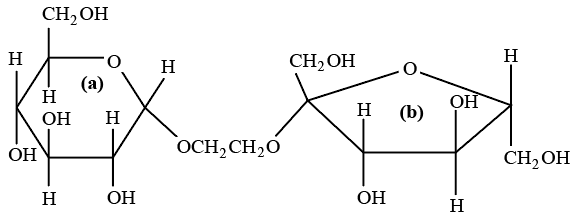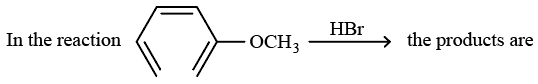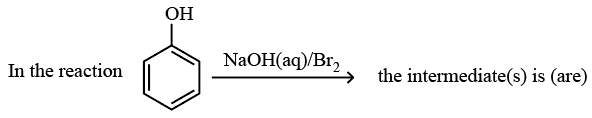Instructions

For the following questions answer them individually

Question 1

# The correct structure of ethylenedianlinetetraacetic acid (EDTA) isQuestion 2

# The ionization ísomer of $$[Cr(H_{2}O)_{4}Cl(NO_{2})]Cl$$ isQuestion 3

# The synthesis of 3-octyne is achieved by adding a bromoalkane into a mixture of sodium amide and an alkyne. The bromoalkane and alkyne respectively areQuestion 4

# The correct statement about the following disaccharide isQuestion 5

#Question 6

# Plots showing the variation of the rate constant (k) with temperature (T) are given below. The plot that follows Arrhenius equation isQuestion 7

# The species which by definition has ZERO standard molar enthalpy of formation at 298 K isQuestion 8

# The bond energy (in kcal $$mol^{-1}$$) of a C-C single bond is approximatelyQuestion 9

# The reagent(s) used for softening the temporary hardness of water is(are)Question 10

#OR